Next: Applications/New Directions Up: Order vs. Algebraic Topology Previous: Triangulations of

## More Late-Breaking News ...

In May-June 1996 results that give algebraic, resp. combinatorial proofs   for the result that every finite truncated noncomplemented lattice has the fixed point property were announced by K. Baclawski in  and by T. McKee and E. Prisner in . We will present McKee and Prisner's approach here as their methods are natural applications of what is discussed in this chapter. The following is thus nothing but a translation of the results in  from their original version for modulo 2 homology to integer homology. It becomes necessary, since the beautiful geometric approach to homology in  seems to depend on the fact that -1=1 in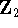, while the arguments generalize beyond this visualization. One just needs to carefully keep track of the signs at times.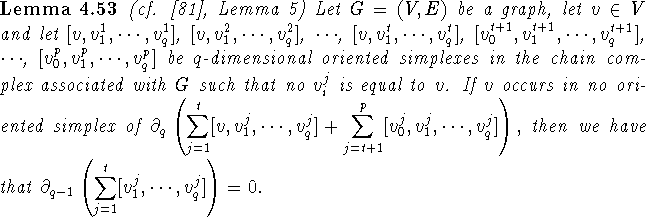Proof: In case q=1, there is nothing to prove (the boundary of a sum of zero-dimensional simplexes is 0). Let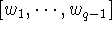be a (q-2)-dimensional oriented simplex that occurs in one of the (q-1)-dimensional simplexes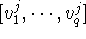, with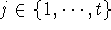. Then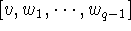occurs once (positive or negative) in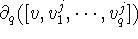, but by assumption it does not occur in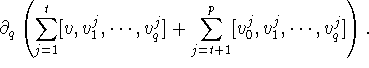Thereforeoccurs in an even number, say 2k, of the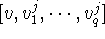() and it occurs such that in k of thethe summandis positive and in the other k boundaries it is negative. Ifis a positive summand of, thenis a negative summand of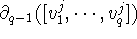and vice versa. Thus no multiple ofoccurs in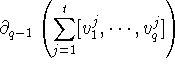by the observed cancellation. The conclusion follows. \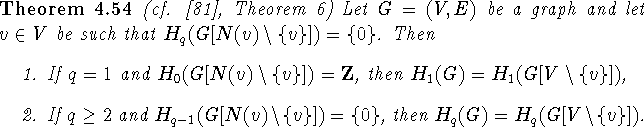Proof: Assume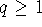,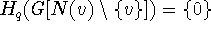. If q=1 assume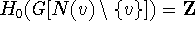, if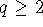assume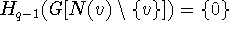.
First, we show that every homology class of q-dimensional cycles has a representative that does not contain v. Assume. Let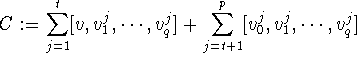be a representative of the homology class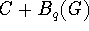, with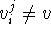. If t=0, we are done, so we can assume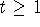. By Lemma 4.53,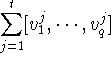is a (q-1)-cycle of G and hence also of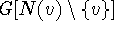. Sincethere are q-simplexes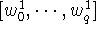,,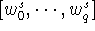ofsuch that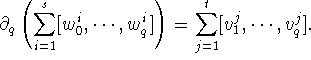But then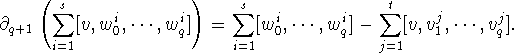Thus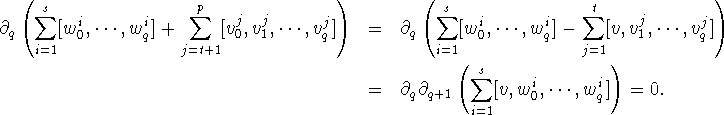Thus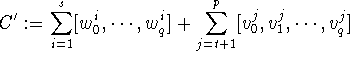forms a q-dimensional cycle with vertices in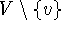. Finally C and C' are homologous, since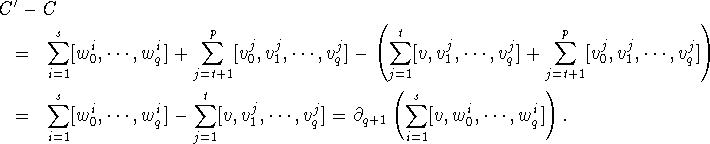In case q=1, let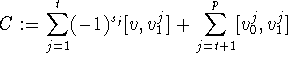be a representative of the homology class, with. t must be even, say t=2k and k of the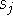must be 0, the rest 1 (otherwise the boundary of C contains a multiple of [v]). Since, we have by Lemma 4.19 thatis connected. However then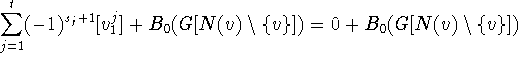, since in a connected graph any sum of differences of zero-dimensional simplexes is the boundary of a sum of 1-dimensional simplexes. Now the argument continues as in the case.
If we can show now that for any q-cycles C,C' that do not contain v we have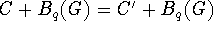iff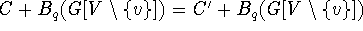then the map that maps every homology class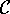in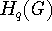to the corresponding homology class in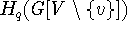defined by the representatives ofthat do not contain v is an isomorphism and we are done. To do so first note that the direction ``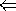" is trivial. For the other direction let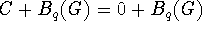with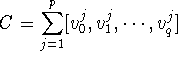with. Then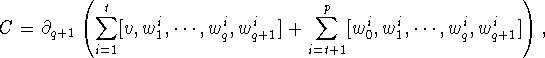with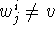. By Lemma 4.53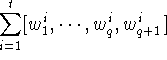is a q-cycle and sincethere must be (q+1)-dimensional simplexes insuch that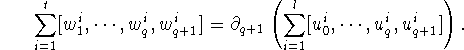But then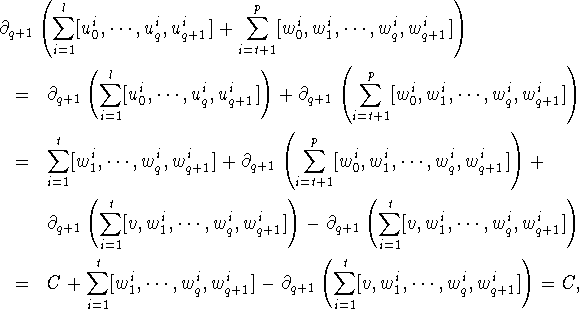with the cancellation in the last step happening sinceis a cycle in. This finishes the proof. \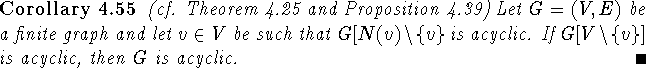This together with the removal of escamotable points as discussed in section 4.5 gives a proof of Baclawski and Björner's result that is entirely algebraic. (Contractibility of the neighborhood was needed to guarantee removability of the point without affecting the homology. The contractibility of escamotable graphs was a nice consequence, which is however stronger than what was needed. A notion ``vertex is weakly escamotable iff its pointed neighborhood is acyclic" is strong enough for our purposes.)
Baclawski's argument (cf. ) proves a result similar to Corollary 4.55 about structures called ``pseudo cones" using neither algebra, nor topology. His fixed point algorithm for pseudo cones does not use any previously known method and as with  we refer the reader to the original.Next: Applications/New Directions Up: Order vs. Algebraic Topology Previous: Triangulations of

Bernd.S.W.Schroder